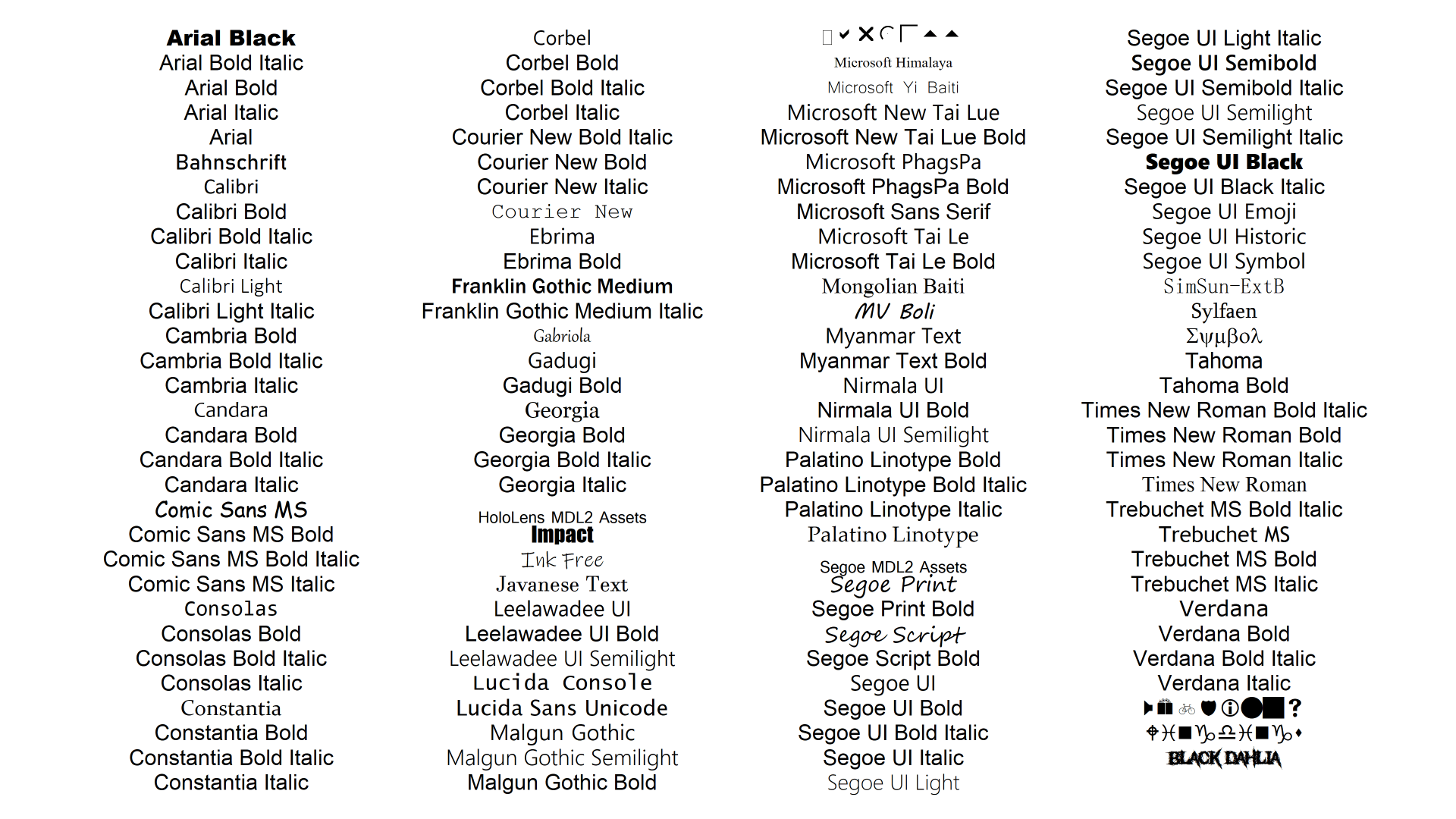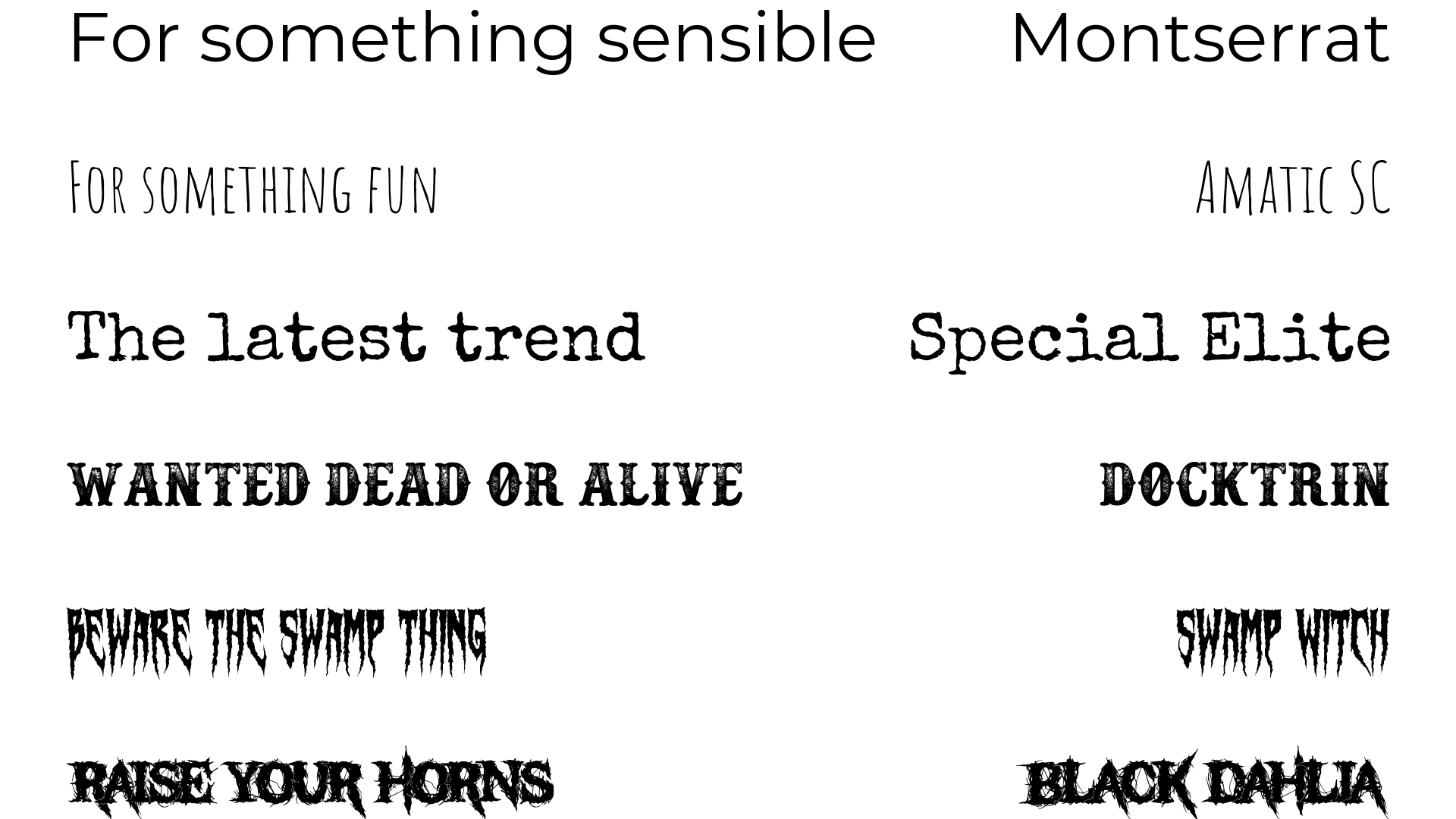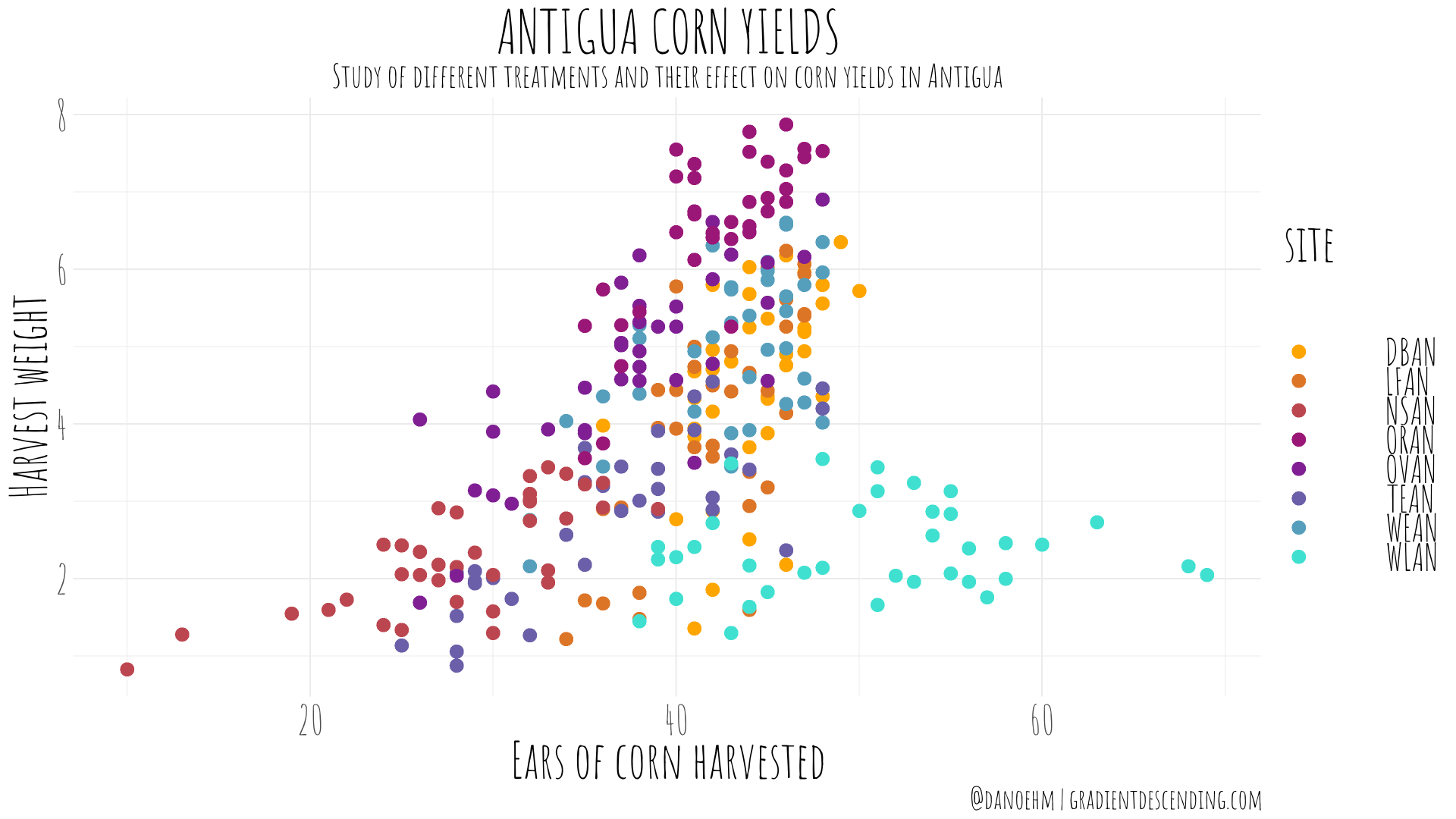Want to share your content on R-bloggers? click here if you have a blog, or here if you don't.ggplot – You can spot one from a mile away, which is great! And when you do it’s a silent fist bump. But sometimes you want more than the standard theme.

Fonts can breathe new life into your plots, helping to match the theme of your presentation, poster or report. This is always a second thought for me and need to work out how to do it again, hence the post.

There are two main packages for managing fonts – `extrafont`, and `showtext`.

## Extrafont

A relatively old package and it’s not well supported unfortunately. You can run into problems, however the base functions work well enough.

The fonts in the system directory are first imported into the `extrafontdb` using `font_import()`. This only needs to be run once in order to load the fonts into the right directory. Secondly, they are registered in R using `loadfonts()` for your specific device. The fonts need to be loaded each session.

```library(tidyverse)
library(extrafont)
library(cowplot)```

```# import fonts - only once
font_import()```

```# load fonts - every session
loadfonts(device = "win", quiet = TRUE)```

Below are all the available fonts (that I have – click on the image to enlarge).To use these fonts in a plot, change the text family using one of the names above. For demonstration I’ll use the Antigua corn data from the DAAG package.

```library(DAAG)

# corn plot
corn <- antigua %>%
dplyr::filter(ears > 0) %>%
ggplot(, aes(x = ears, y = harvwt, col = site)) +
geom_point(size = 4) +
scale_colour_manual(values = colorRampPalette(c("orange", "darkmagenta", "turquoise"))(8)) +
labs(title = "ANTIGUA CORN YIELDS",
x = "Ears of corn harvested",
y = "Harvest weight") +
theme(
text = element_text(family = "candara", size = 24),
plot.title = element_text(size = 30),
plot.caption = element_text(size = 28))
corn```It’s likely you’ll want more than what is available in the standard font set. You can add custom fonts with `extrafont()`, however I’ve only had limited success. A better option is using `showtext`.

## Showtext

`showtext` is a package by Yixuan Qiu and it makes adding new fonts simple. There are a tonne of websites where you can download free fonts to suit pretty much any style you are going for. I’ll only touch on the key bits, so check out the vignette for more details.

The simplest way is to add fonts is via `font_add_google()`. Find the font you like on Google Fonts and add it to R using the following.

```library(showtext)

Amatic SC can now be used by changing the font family to “amatic-sc”. For R to know how to properly render the text we first need to run `showtext_auto()` prior to displaying the plot. One downside is it currently does not display in Rstudio. Either open a new graphics window with `windows()` or save as an external file e.g. `.png`.

```# turn on showtext
showtext_auto()```

Custom fonts are added by first,

• Finding the font you want to use (I’ve picked a few from 1001 Free Fonts and Font Space, but there are many more out there)
• Download the font `.ttf()` file and unzip if needed
• Use `font_add()` to register the font
• Run `showtext_auto()` to load the fonts

```font_add(family = "docktrin", regular = "./fonts/docktrin/docktrin.ttf")
showtext_auto()```

And that’s pretty much it. Given how effortless it is to add new fonts you can experiment with many different styles.These fonts are outrageous but demonstrate that you really can go for any style, from something minimal and easy reading to something fit for a heavy metal band. For professional reports you’ll want to go for something sensible, but if you’re making a poster, website or infographic you may want to get creative e.g.The tools are there for you to be as creative as you want to be.

The last thing to note is you’ll need to play around with different sizes given the resolution of your screen.

To turn off `showtext` and use the standard fonts, run

`showtext_auto(FALSE)`

## Code bits

```# load fonts
ft <- data.frame(x = sort(rep(1:4, 31))[1:nrow(fonttable())], y = rep(31:1, 4)[1:nrow(fonttable())], text_name = fonttable()\$FullName)
font_plot <- ggplot(ft, aes(x = x, y = y)) +
geom_text(aes(label = text_name, family = text_name), size = 20) +
coord_cartesian(xlim = c(0.5, 4.5)) +
theme_void()
font_plot

# amatic sc
scale <- 4.5 # scale is an adjustment for a 4k screen
corn <- ggplot(antigua %>% dplyr::filter(ears > 0), aes(x = ears, y = harvwt, col = site)) +
geom_point(size = 4) +
scale_colour_manual(values = colorRampPalette(c("orange", "darkmagenta", "turquoise"))(8)) +
labs(title = "ANTIGUA CORN YIELDS",
subtitle = "Study of different treatments and their effect on corn yields in Antigua",
x = "Ears of corn harvested",
y = "Harvest weight",
caption = "@danoehm | gradientdescending.com") + theme_minimal() +
theme(
text = element_text(family = "amatic-sc", size = 22*scale),
plot.title = element_text(size = 26*scale, hjust = 0.5),
plot.subtitle = element_text(size = 14*scale, hjust = 0.5),
plot.caption = element_text(size = 12*scale),
legend.text = element_text(size = 16*scale))
png(file = "./fonts/corn yields amatic-sc.png", width = 3840, height = 2160, units = "px", res = 72*4)
corn
dev.off()

# generate data - unfortunately I couldn't find data on actual outlaws
n <- 20
x <- 100*runif(n)
y <- (25 + 0.005*x^2 + rnorm(n, 0, 10))*10
wanted <- data.frame(x, y) %>%
ggplot(aes(x = x, y = y)) +
geom_smooth(col = "black", lty = 2) +
geom_point(size = 4) +
theme_minimal() +
labs(title = "WANTED: DEAD OR ALIVE",
subtitle = "Relationship of the crimes committed by outlaws and the bounty on their head",
x = "CRIMES",
y = "BOUNTY",
caption = "@danoehm | gradientdescending.com") + theme_minimal() +
theme(
text = element_text(family = "docktrin", size = 16*scale),
plot.title = element_text(size = 40*scale, hjust = 0.5),
plot.subtitle = element_text(size = 14*scale, hjust = 0.5, margin = margin(t = 40)),
plot.caption = element_text(size = 10*scale),
legend.text = element_text(size = 12*scale),
panel.grid = element_line(color = "black"),
axis.title = element_text(size = 26*scale),
axis.text = element_text(color = "black"))
png(file = "./fonts/wanted1.png", width = 3840, height = 2160, units = "px", res = 72*4)
ggdraw() +
draw_image("./fonts/wanted_dead_or_alive_copped.png", scale = 1.62) + # a png of the background for the plot
draw_plot(wanted)
dev.off()

# Horror movies
gghorror <- horror %>%
dplyr::filter(str_detect(keywords, "horror")) %>%
dplyr::select(original_title, release_date, vote_average) %>%
ggplot(aes(x = release_date, y = vote_average)) +
geom_smooth(col = "#003300", lty = 2) +
geom_point(size = 4, col = "white") +
labs(title = "HORROR MOVIES!",
subtitle = "Average critics ratings of horror films and relationship over time",
x = "YEAR",
y = "RATING",
caption = "Data from IMDB.\n@danoehm | gradientdescending.com") + theme_minimal() +
theme(
text = element_text(family = "swamp-witch", size = 16*scale, color = "#006600"),
plot.title = element_text(size = 48*scale, hjust = 0.5),
plot.subtitle = element_text(family = "montserrat", size = 14*scale, hjust = 0.5, margin = margin(t = 30)),
plot.caption = element_text(family = "montserrat", size = 8*scale),
legend.text = element_text(size = 12*scale),
panel.grid = element_line(color = "grey30"),
axis.title = element_text(size = 26*scale),
axis.title.y = element_text(margin = margin(r = 15)),
axis.text = element_text(color = "#006600"),
plot.background = element_rect(fill = "grey10"))
png(file = "./fonts/swamp.png", width = 3840, height = 2160, units = "px", res = 72*4)
gghorror
dev.off()```

The post Adding Custom Fonts to ggplot in R appeared first on Daniel Oehm | Gradient Descending.